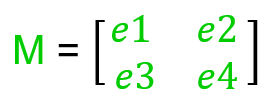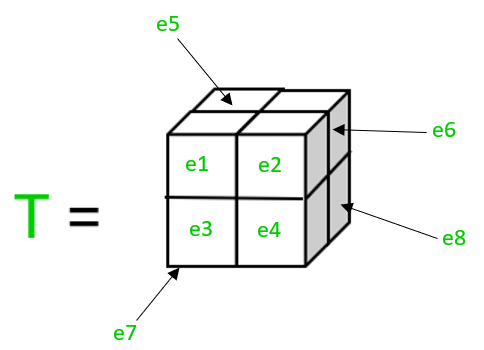# ML | Linear Algebra Operations

Linear Algebra principles are crucial for understanding the concept behind Machine Learning, as well as Deep Learning, even many ideas struggle to create a precise mathematical model, linear algebra continues to be an important tool for researching them. Algebra gives you a better understanding of how algorithms actually operate under the hood so a developer can make better choices and one can’t escape learning some of these techniques if one wants to be a professional in Machine Learning or Deep Learning.

What is Linear Algebra ?
It is a branch of mathematics that allows to define and perform operations on higher-dimensional coordinates and plane interactions in a concise way. Linear Algebra is an algebra extension to an undefined number of dimensions. Linear Algebra concerns the focus on linear equation systems. It is a continuous type of mathematics and is applicable in science and engineering, as it helps one to model and efficiently simulate natural phenomena.

Before progressing to Linear Algebra concepts, we must understand the below properties:

• Associative Property: It is a property in Mathematics which states that if a, b and c are mathematical objects than a + (b + c) = (a + b) + c in which + is a binary operation.
• Commutative Property: It is a property in Mathematics which states that if a and b are mathematical objects then a + b = b + a in which + is a binary operation.
• Distributive Property: It is a property in Mathematics which states that if a, b and c are mathematical objects then a * (b + c)= (a * b) + (a * c) in which * and + are binary operators.

Below are some linear Algebra concepts which are mostly used in Machine Learning implementation:

• Scalar: It is a physical quantity described using a single element, It has only magnitude and not direction. Basically, a scalar is just a single number.
```Example:
17 and 256```
• Vector: It is a geometric object having both magnitude and direction, it is an ordered number array, and are always in a row or column. A Vector has just one index, which can refer to a particular value within the Vector.Here V is a vector in which e1, e2, e3 and e4 are its elements, and V is e3.

```Example:
[2, 3, 4] and [34, 53]```

Operations:

• Scalar-Vector Multiplication:
`p = [e1, e2, e3]`

The product of a scalar with a vector gives the below result-

`p * 2 = [2 * e1, 2 * e2, 2 * e3]`

when the scalar 2 is multiplied by a vector p then all the elements of the vector p is multiplied by that scalar. This operation satisfies commutative property.

```Example:
x = [1, 2]
x * 4 = [4, 8]```
• Matrix: It is an ordered 2D array of numbers, symbols or expressions arranged in rows and columns. It has two indices, the first index points to the row, and the second index points to the column. A Matrix can have multiple numbers of rows and columns.Above M is a 2D matrix having e1, e2, e3 and e4 as elements, and M is e3.

```Example:
2 3 6  and 56 12
4 5 8      45 78
34 67 ```

A matrix having its left diagonal elements as 1 and other elements 0 is an Identity matrix.

```Example:
1 0
0 1 is 2D Identity Matrix.

1 0 0
0 1 0
0 0 1 is 3D Identity Matrix.```

Operations:

• Scalar-Matrix Multiplication:
```p = e1 e2
e3 e4
a is a scalar.
p * a = (a * e1)  (a * e2)
(a * e3)  (a * e4)```

When the scalar a is multiplied by a matrix p then all the elements of the matrix p is multiplied by that scalar. Scalar-Matrix multiplication is associative, distributive and commutative.

```Example:
x = 1 2 3
4 5 6
x * 4 = 4  8  12
16 20 24```
• Vector-Matrix Multiplication:
```p = e1 e2
e3 e4
e5 e6
q = a
b```

Multiplying a matrix p with a vector q gives the below product-

```p * q = (e1 * a) + (e2 * b)
(e3 * a) + (e4 * b)
(e5 * a) + (e6 * b)```

The number of rows of a matrix should be equal to the number of elements of the vector then only they can be multiplied. Vector-Matrix multiplication is associative and distributive but not commutative.

```Example:
m = 1 2  and n = 1
3 4          2
5 6
then m * n = 5
11
17```
```m1 = a b  and m2 = p q
c d           r s```

In-order to add matrices the rows and columns of the matrices should be equal. The addition of matrix m1 and m2 gives the below result-

```m1 + m2 = (a + p) (b + q)
(c + r) (d + s)```

Each element of the first matrix is added with the respective element of the another matrix both having same row and column value. Matrix-Matrix addition is associative, distributive and commutative.

```Example:
1 2   5 5   6 7
2 1 + 5 5 = 7 6
1 2   5 5   6 7 ```
• Matrix-Matrix Subtraction:
```m1 = a b  and m2 = p q
c d           r s```

In-order to subtract matrices, the rows and columns of the matrices should be equal. The subtraction between matrix m1 and m2 gives the below result-

```m1 - m2 = (a - p) (b - q)
(c - r) (d - s)```

Each element of the first matrix is subtracted with the respective element of the another matrix both having same row and column value. Matrix-Matrix addition is associative, distributive and commutative.

```Example:
1 2   5 5   -4 -3
2 1 - 5 5 = -3 -4
1 2   5 5   -4 -3```
• Matrix-Matrix Multiplication:
```m1 = a b  and m2 = p q
c d           r s```

To multiply two matrices, the number of columns of the first matrix should be equal to the number of rows in the second matrix, the product of matrix m1 and m2 is given below-

```m1 * m2 = ((a * p) + (b * r))   ((a * q) + (b * s))
((c * p) + (d * r))   ((c * q) + (d * s))```

Matrix-Matrix multiplication is associative and distributive but not commutative.

```Example:
1 3 2   1 3    11 10
4 0 1 * 0 1 =  9  14
5 2
1 3 2   1    11
4 0 1 * 0 =  9
5
1 3 2   3    10
4 0 1 * 1 =  14
2 ```

Let x1, x2 and x3 be matrices such that x1 has a number of rows and b number of columns and x2 has b number of rows and c number of columns and x3 is the product of x1 and x2 so, x3 will have a number of rows and c number of columns. x1 * x2 = x3 in which x3 has a rows and c columns.

• Transpose:
The transpose of a matrix generates a new matrix in which the rows become columns and columns become rows of the original matrix.

```m = a b
c d
Transpose(m) = a c
b d  ```

If A is a matrix and B is the transpose of matrix A then, transpose of matrix B is the original matrix A.
B = Transpose(A) then, Transpose(B)=Transpose(Transpose(A)) = A

```Example:
x = 1 2 3
Transpose of matrix x is 1
2
3```

Transpose of an m*n matrix will give a n*m matrix.

• Inverse:
The inverse of a matrix is the matrix when multiplied with the original matrix gives the Identity matrix as the product. If m is a matrix and n is the inverse matrix of m, then m*n = I, in which I represent Identity matrix.

```Example:
m = 4 7 and inverse(m) =  0.6 -0.7
2 6                  -0.2 0.4
4 7  *  0.6 -0.7 = 1 0
2 6    -0.2  0.4   0 1 ```
• Tensor: It is an algebraic object representing a linear mapping of algebraic objects from one set to another. It is actually a 3D array of numbers with a variable number of axes, arranged on a regular grid. A tensor has three indices, first index points to the row, the second index points to the column and the third index points to the axis.Here the tensor T has 8 elements e1, e2, e3, e4, e5, e6, e7 and e8 in which T is e8 .

My Personal Notes arrow_drop_upCheck out this Author's contributed articles.

If you like GeeksforGeeks and would like to contribute, you can also write an article using contribute.geeksforgeeks.org or mail your article to contribute@geeksforgeeks.org. See your article appearing on the GeeksforGeeks main page and help other Geeks.

Please Improve this article if you find anything incorrect by clicking on the "Improve Article" button below.